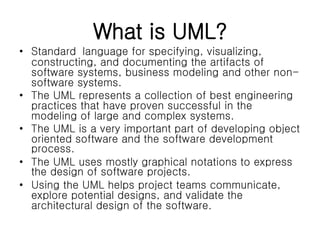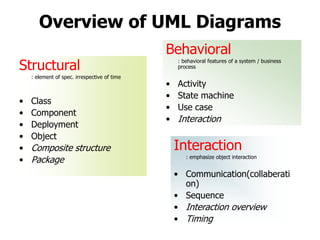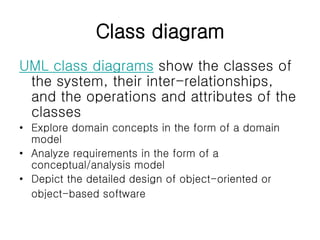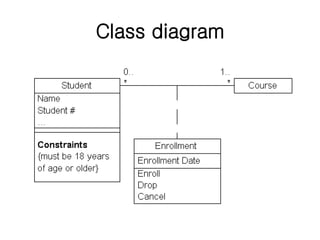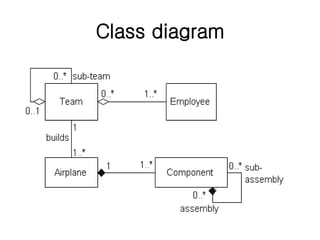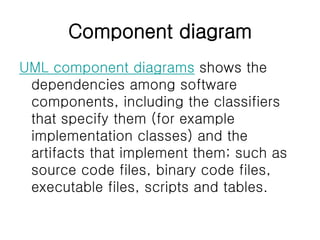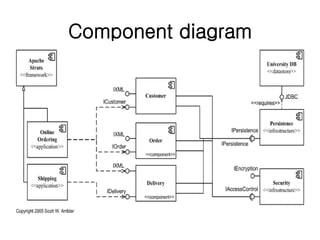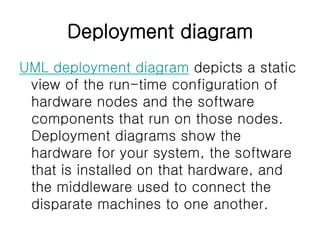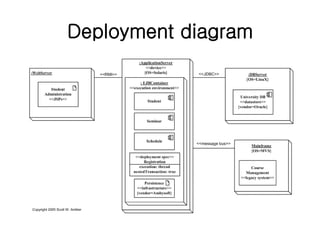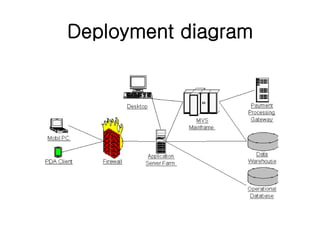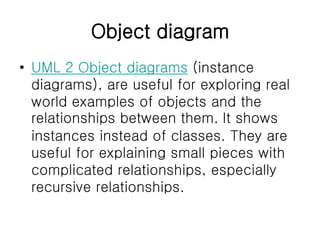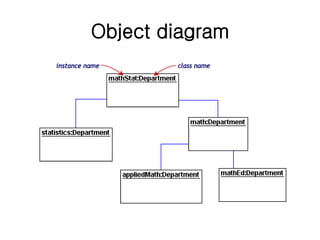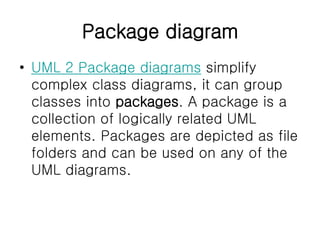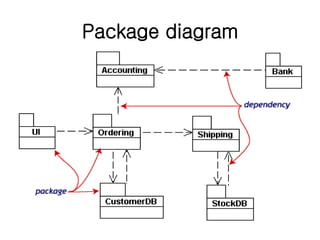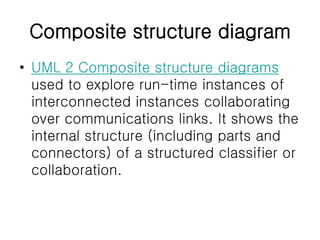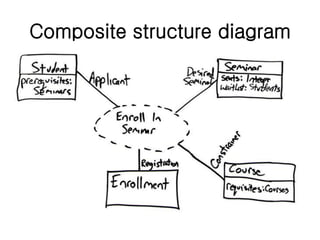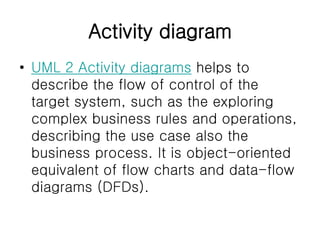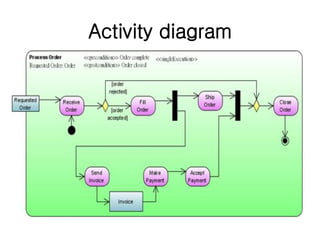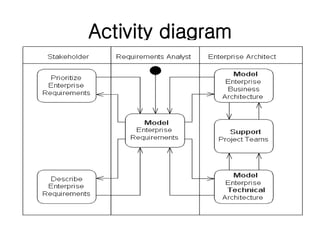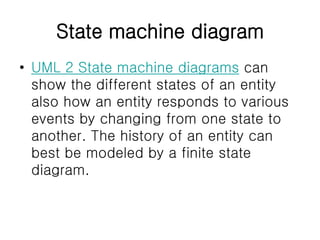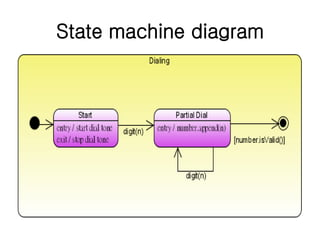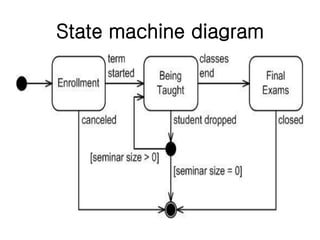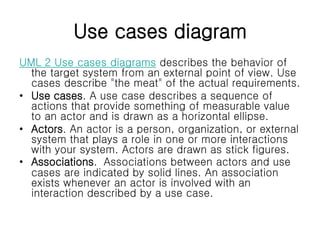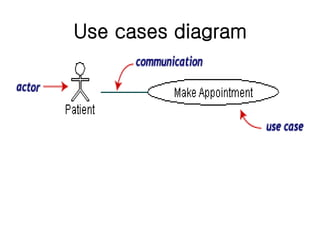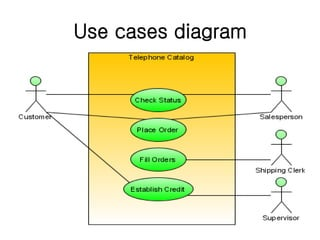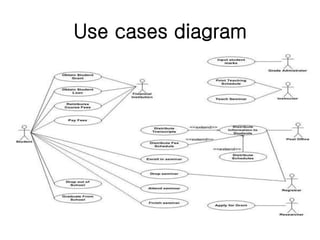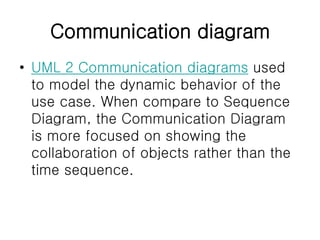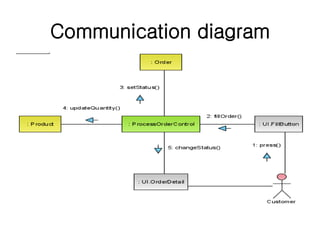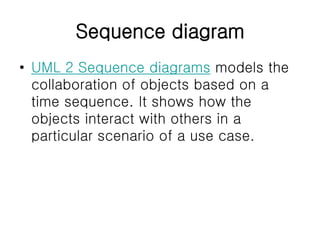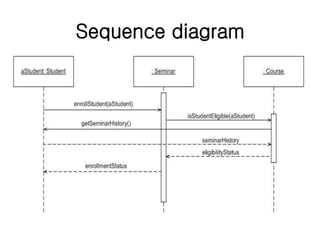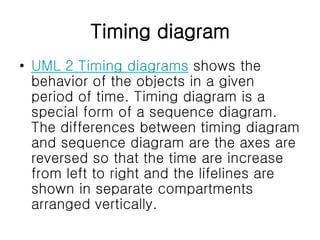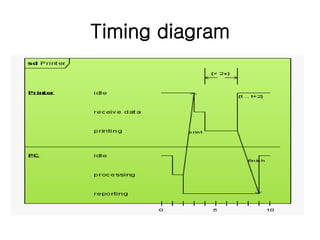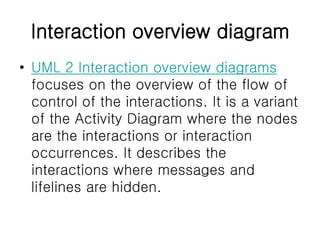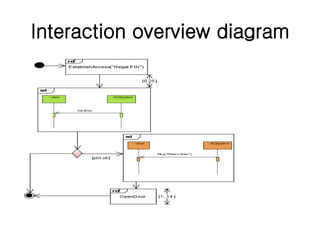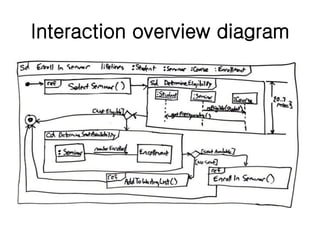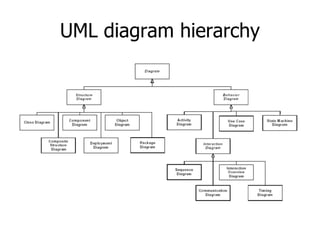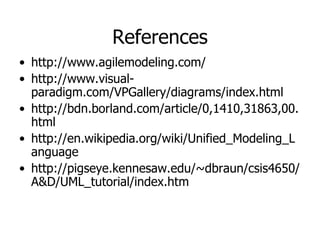1 de 39

### Lect-4: UML diagrams - Unified Modeling Language - SPM

1. UML Diagrams Lecture-4 Mr. Mubashir Ali Lecturer(Dept. of Computer Science) dr.mubashirali1@gmail.com
2. What is UML? • Standard language for specifying, visualizing, constructing, and documenting the artifacts of software systems, business modeling and other non- software systems. • The UML represents a collection of best engineering practices that have proven successful in the modeling of large and complex systems. • The UML is a very important part of developing object oriented software and the software development process. • The UML uses mostly graphical notations to express the design of software projects. • Using the UML helps project teams communicate, explore potential designs, and validate the architectural design of the software.
3. Overview of UML Diagrams Structural : element of spec. irrespective of time • Class • Component • Deployment • Object • Composite structure • Package Behavioral : behavioral features of a system / business process • Activity • State machine • Use case • Interaction Interaction : emphasize object interaction • Communication(collaberati on) • Sequence • Interaction overview • Timing
4. Class diagram UML class diagrams show the classes of the system, their inter-relationships, and the operations and attributes of the classes • Explore domain concepts in the form of a domain model • Analyze requirements in the form of a conceptual/analysis model • Depict the detailed design of object-oriented or object-based software
5. Class diagram
6. Class diagram
7. Component diagram UML component diagrams shows the dependencies among software components, including the classifiers that specify them (for example implementation classes) and the artifacts that implement them; such as source code files, binary code files, executable files, scripts and tables.
8. Component diagram
9. Deployment diagram UML deployment diagram depicts a static view of the run-time configuration of hardware nodes and the software components that run on those nodes. Deployment diagrams show the hardware for your system, the software that is installed on that hardware, and the middleware used to connect the disparate machines to one another.
10. Deployment diagram
11. Deployment diagram
12. Object diagram • UML 2 Object diagrams (instance diagrams), are useful for exploring real world examples of objects and the relationships between them. It shows instances instead of classes. They are useful for explaining small pieces with complicated relationships, especially recursive relationships.
13. Object diagram
14. Package diagram • UML 2 Package diagrams simplify complex class diagrams, it can group classes into packages. A package is a collection of logically related UML elements. Packages are depicted as file folders and can be used on any of the UML diagrams.
15. Package diagram
16. Composite structure diagram • UML 2 Composite structure diagrams used to explore run-time instances of interconnected instances collaborating over communications links. It shows the internal structure (including parts and connectors) of a structured classifier or collaboration.
17. Composite structure diagram
18. Activity diagram • UML 2 Activity diagrams helps to describe the flow of control of the target system, such as the exploring complex business rules and operations, describing the use case also the business process. It is object-oriented equivalent of flow charts and data-flow diagrams (DFDs).
19. Activity diagram
20. Activity diagram
21. State machine diagram • UML 2 State machine diagrams can show the different states of an entity also how an entity responds to various events by changing from one state to another. The history of an entity can best be modeled by a finite state diagram.
22. State machine diagram
23. State machine diagram
24. Use cases diagram UML 2 Use cases diagrams describes the behavior of the target system from an external point of view. Use cases describe "the meat" of the actual requirements. • Use cases. A use case describes a sequence of actions that provide something of measurable value to an actor and is drawn as a horizontal ellipse. • Actors. An actor is a person, organization, or external system that plays a role in one or more interactions with your system. Actors are drawn as stick figures. • Associations. Associations between actors and use cases are indicated by solid lines. An association exists whenever an actor is involved with an interaction described by a use case.
25. Use cases diagram
26. Use cases diagram
27. Use cases diagram
28. Communication diagram • UML 2 Communication diagrams used to model the dynamic behavior of the use case. When compare to Sequence Diagram, the Communication Diagram is more focused on showing the collaboration of objects rather than the time sequence.
29. Communication diagram
30. Sequence diagram • UML 2 Sequence diagrams models the collaboration of objects based on a time sequence. It shows how the objects interact with others in a particular scenario of a use case.
31. Sequence diagram
32. Timing diagram • UML 2 Timing diagrams shows the behavior of the objects in a given period of time. Timing diagram is a special form of a sequence diagram. The differences between timing diagram and sequence diagram are the axes are reversed so that the time are increase from left to right and the lifelines are shown in separate compartments arranged vertically.
33. Timing diagram
34. Timing diagram
35. Interaction overview diagram • UML 2 Interaction overview diagrams focuses on the overview of the flow of control of the interactions. It is a variant of the Activity Diagram where the nodes are the interactions or interaction occurrences. It describes the interactions where messages and lifelines are hidden.
36. Interaction overview diagram
37. Interaction overview diagram
38. UML diagram hierarchy
39. References • http://www.agilemodeling.com/ • http://www.visual- paradigm.com/VPGallery/diagrams/index.html • http://bdn.borland.com/article/0,1410,31863,00. html • http://en.wikipedia.org/wiki/Unified_Modeling_L anguage • http://pigseye.kennesaw.edu/~dbraun/csis4650/ A&D/UML_tutorial/index.htm